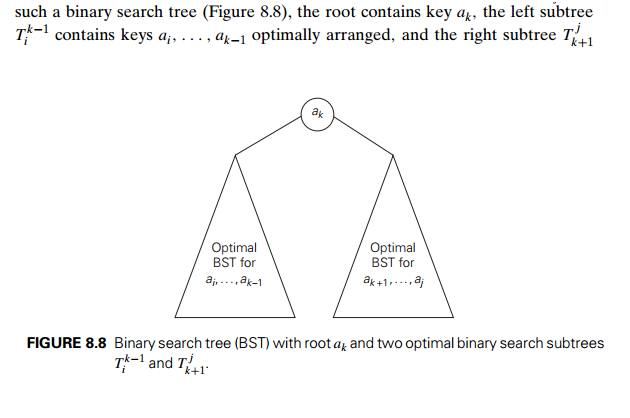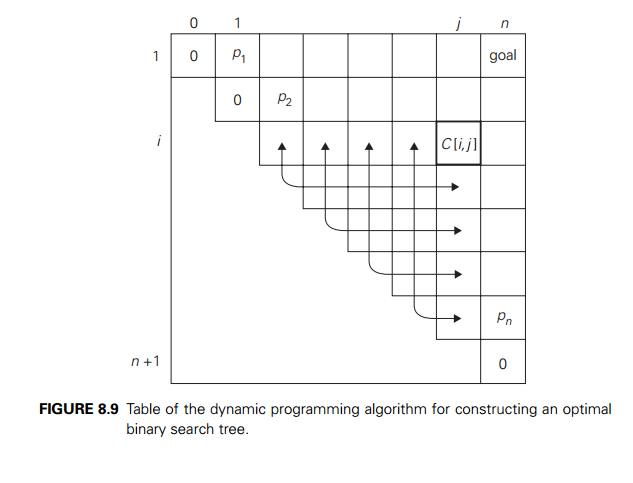Home | | Design and Analysis of Algorithms | Optimal Binary Search Trees

# Optimal Binary Search Trees

A binary search tree is one of the most important data structures in computer science. One of its principal applications is to implement a dictionary, a set of elements with the operations of searching, insertion, and deletion.

Optimal Binary Search Trees

A binary search tree is one of the most important data structures in computer science. One of its principal applications is to implement a dictionary, a set of elements with the operations of searching, insertion, and deletion. If probabilitiesof searching for elements of a set are knownŌĆöe.g., from accumulated data about past searchesŌĆöit is natural to pose a question about an optimal binary search tree for which the average number of comparisons in a search is the smallest possible. For simplicity, we limit our discussion to minimizing the average number of comparisons in a successful search. The method can be extended to include unsuccessful searches as well.

As an example, consider four keys A, B, C, and D to be searched for with probabilities 0.1, 0.2, 0.4, and 0.3, respectively. Figure 8.7 depicts two out of 14 possible binary search trees containing these keys. The average number of

comparisons in a successful search in the first of these trees is 0.1 . 1 + 0.2 . 2 + 0.4 . 3 + 0.3 . 4 = 2.9, and for the second one it is 0.1 . 2 + 0.2 . 1 + 0.4 . 2 + 0.3 . 3 = 2.1.

Neither of these two trees is, in fact, optimal. (Can you tell which binary tree is optimal?)

For our tiny example, we could find the optimal tree by generating all 14 binary search trees with these keys. As a general algorithm, this exhaustive-search approach is unrealistic: the total number of binary search trees with n keys is equal to the nth Catalan number,which grows to infinity as fast as 4n/n1.5 (see Problem 7 in this sectionŌĆÖs exercises). So let a1, . . . , an be distinct keys ordered from the smallest to the largest and let p1, . . . , pn be the probabilities of searching for them. Let C(i, j ) be the smallest average number of comparisons made in a successful search in a binary search tree Tij made up of keys ai, . . . , aj , where i, j are some integer indices, 1 Ōēż i Ōēż j Ōēż n. Following the classic dynamic programming approach, we will find values of C(i, j ) for all smaller instances of the problem, although we are interested just in C(1, n). To derive a recurrence underlying a dynamic programming algorithm, we will consider all possible ways to choose a root ak among the keys ai, . . . , aj . For such a binarycontains keys ak+1, . . . , aj also optimally arranged. (Note how we are taking advantage of the principle of optimality here.)

If we count tree levels starting with 1 to make the comparison numbers equal the keysŌĆÖ levels, the following recurrence relation is obtained:We assume in formula (8.8) that C(i, i ŌłÆ 1) = 0 for 1 Ōēż i Ōēż n + 1, which can be interpreted as the number of comparisons in the empty tree. Note that this formula implies that

C(i, i) = pi                             for 1 Ōēż i Ōēż n,

as it should be for a one-node binary search tree containing ai.The two-dimensional table in Figure 8.9 shows the values needed for comput-ing C(i, j ) by formula (8.8): they are in row i and the columns to the left of column j and in column j and the rows below row i. The arrows point to the pairs of en-tries whose sums are computed in order to find the smallest one to be recorded as the value of C(i, j ). This suggests filling the table along its diagonals, starting with all zeros on the main diagonal and given probabilities pi, 1 Ōēż i Ōēż n, right above it and moving toward the upper right corner.

The algorithm we just sketched computes C(1, n)ŌĆöthe average number of comparisons for successful searches in the optimal binary tree. If we also want to get the optimal tree itself, we need to maintain another two-dimensional table to record the value of k for which the minimum in (8.8) is achieved. The table has the same shape as the table in Figure 8.9 and is filled in the same manner, starting with entries R(i, i) = i for 1 Ōēż i Ōēż n. When the table is filled, its entries indicate indices of the roots of the optimal subtrees, which makes it possible to reconstruct an optimal tree for the entire set given.

EXAMPLE Let us illustrate the algorithm by applying it to the four-key set we used at the beginning of this section:Thus, out of two possible binary trees containing the first two keys, A and B, the root of the optimal tree has index 2 (i.e., it contains B), and the average number of comparisons in a successful search in this tree is 0.4.

We will ask you to finish the computations in the exercises. You should arrive at the following final tables:Thus, the average number of key comparisons in the optimal tree is equal to 1.7. Since R(1, 4) = 3, the root of the optimal tree contains the third key, i.e., C. Its left subtree is made up of keys A and B, and its right subtree contains just key D (why?). To find the specific structure of these subtrees, we find first their roots by consulting the root table again as follows. Since R(1, 2) = 2, the root of the optimal tree containing A and B is B, with A being its left child (and the root of the one-node tree: R(1, 1) = 1). Since R(4, 4) = 4, the root of this one-node optimal tree is its only key D. Figure 8.10 presents the optimal tree in its entirety.Here is pseudocode of the dynamic programming algorithm.

ALGORITHM     OptimalBST(P [1..n])

//Finds an optimal binary search tree by dynamic programming

//Input: An array P [1..n] of search probabilities for a sorted list of n keys //Output: Average number of comparisons in successful searches in the // optimal BST and table R of subtreesŌĆÖ roots in the optimal BST for i ŌåÉ 1 to n do

C[i, i ŌłÆ 1] ŌåÉ 0 C[i, i] ŌåÉ P [i] R[i, i] ŌåÉ i

C[n + 1, n] ŌåÉ 0

for d ŌåÉ 1 to n ŌłÆ 1 do //diagonal count for i ŌåÉ 1 to n ŌłÆ d do

j ŌåÉ i + d minval ŌåÉ Ōł×

for k ŌåÉ i to j do

if C[i, k ŌłÆ 1] + C[k + 1, j ] < minval

minval ŌåÉ C[i, k ŌłÆ 1] + C[k + 1, j ]; kmin ŌåÉ k R[i, j ] ŌåÉ kmin

sum ŌåÉ P [i]; for s ŌåÉ i + 1 to j do sum ŌåÉ sum + P [s] C[i, j ] ŌåÉ minval + sum

return C[1, n], R

The algorithmŌĆÖs space efficiency is clearly quadratic; the time efficiency of this version of the algorithm is cubic (why?). A more careful analysis shows that entries in the root table are always nondecreasing along each row and column. This limits values for R(i, j ) to the range R(i, j ŌłÆ 1), . . . , R(i + 1, j ) and makes it possible to reduce the running time of the algorithm to  (n2).

Exercises 8.3

Finish the computations started in the sectionŌĆÖs example of constructing an optimal binary search tree.

a.  Why is the time efficiency of algorithm OptimalBST cubic?

b. Why is the space efficiency of algorithm OptimalBST quadratic?

Write pseudocode for a linear-time algorithm that generates the optimal binary search tree from the root table.

4.  Devise a way to compute the sums s=i ps, which are used in the dynamic programming algorithm for constructing an optimal binary search tree, in constant time (per sum).

True or false: The root of an optimal binary search tree always contains the key with the highest search probability?

How would you construct an optimal binary search tree for a set of n keys if all the keys are equally likely to be searched for? What will be the average number of comparisons in a successful search in such a tree if n = 2k?

a. Show that the number of distinct binary search trees b(n) that can be constructed for a set of n orderable keys satisfies the recurrence relationIt is known that the solution to this recurrence is given by the Catalan numbers. Verify this assertion for n = 1, 2, . . . , 5.

Find the order of growth of b(n). What implication does the answer to this question have for the exhaustive-search algorithm for constructing an optimal binary search tree?

Design a  (n2) algorithm for finding an optimal binary search tree.

Generalize the optimal binary search algorithm by taking into account unsuc-cessful searches.

Write pseudocode of a memory function for the optimal binary search tree problem. You may limit your function to finding the smallest number of key comparisons in a successful search.

Matrix chain multiplication Consider the problem of minimizing the total number of multiplications made in computing the product of n matricesGive an example of three matrices for which the number of multiplications in (A1 . A2) . A3 and A1 . (A2 . A3) differ at least by a factor of 1000.

How many different ways are there to compute the product of n matrices?

Design a dynamic programming algorithm for finding an optimal order of multiplying n matrices.

Study Material, Lecturing Notes, Assignment, Reference, Wiki description explanation, brief detail
Introduction to the Design and Analysis of Algorithms : Dynamic Programming : Optimal Binary Search Trees |

Related Topics